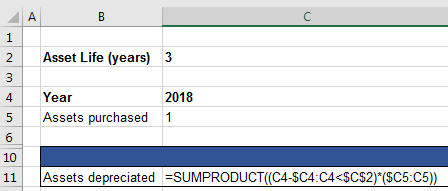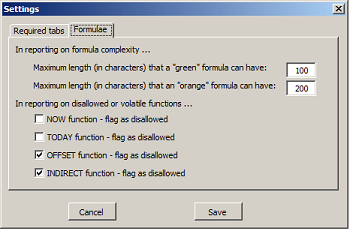See a video of how to use our spreadsheet quality checker

#XL-Excellence® - Examples - Formula functions

Some spreadsheet functions cause heavy calculation loads and often can be replaced by faster functions. The XL-Excellence® Add-In can help in detecting and reporting such functions.

Consider the following example.

The spreadsheet shown below deals with depreciation: Assets are purchased in each year. And they depreciate over a period defined in cell C2. Currently assets depreciate over three years.Row 8 calculates, as at the end of each year, the amount of assets that have depreciated over that year.

Since asset life is currently three years then – for each year – the calculation must add the value of assets purchased over that year and the prior two years. If, however, C2 was 4 and assets depreciated over four years then the calculation must refer to the current and prior three years.

Shown below is an XL-Excellence® report that assesses two alternative ways of performing the depreciating assets calculation.The heatmap above has been generated to report on formula functions – whether or not they are on a “do-not-use” register. The calculations on row 8 – shown in red – use a function (OFFSET) that the user nominated as being "disallowed".The alternative calculations on row 11 use “allowed” functions.You can set which are “allowed” functions and “disallowed” by using the settings option ..Why is the OFFSET function best avoided if an alternative is available? Because it is a “volatile” function that causes a high computer load when any cell changes in any open workbook.

If you wish to experiment with the examples discussed above you can download the examples by choosing from the Links section.